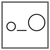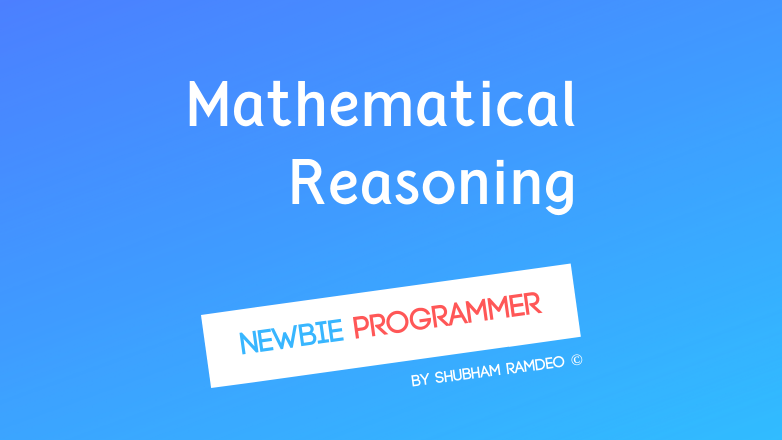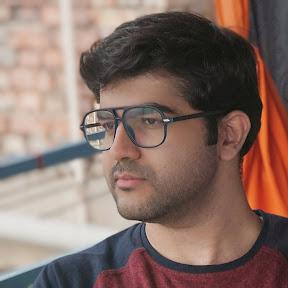Fuddlepixel coz tech is fun();

# Mathematical Reasoning

Let us discuss how maths can perform logical operations and not only calculations. It is the subject called mathematical reasoning to do logic in maths !

By Shubham Ramdeo
06 September 2015 · 5 mins read
# `newbie`  `maths`Maths is not only for addition and subtraction, it can really do have logical sense ! Mathematical Reasoning can do that !

Mathematics ! Some of you might feel excited as we are discussing some Mathematics at a Programming Blog. While I believe most of you will hate and say, maths here too ?!?!

Well the truth is, yes ! Maths is everywhere. Even in Logic and Reasoning. And that’s what we are gonna discuss today.  As always, this miscellaneous post is not intended, its gonna be useful before going into Bitwise Operators of our Newbie Programmer Series. But I think you will like this introduction to a new form of mathematics. Before reading this, I will recommend you to read a recent post of mine about Electronic Logic (click here). That will help you a lot to understand all this.

Mathematical Reasoning is a branch of applied mathematics. That means here we apply mathematics to make our work done. With Mathematical Reasoning, we can use mathematics over logical analysis.

We will discuss only those simple things which are useful to Newbie Programmer Series and will skip the advanced parts

## The Statements

Just as we did in a recent post “The Electronic Logic” (click here) Here too, we have three things :

• If a statement is logically correct, its TRUE (T) (we can say 1)
• If a statement is logically incorrect, its FALSE (F) (we can say 0)
• The most important thing is the definition of Statement, A statement is like an information, an answer. It can either be True or False but can never both.

For example :

• p : “Sun rises in the east.” It is a True Statement. So ( 1 )
• q : “Moon looks Orange.” It is a False Statement. So ( 0 )
• r : “How beautiful the sky is !” It is not a statement.

### Truth Tables

This is a very simple thing. Here we simply write Combinations of T if True and F if False for the statements to analyse them.

So as above we have three statements, p, q and r.

Suppose we want to make Combinations of statements p and q then :

``````------
p    q
------
T   T
T   F
F   T
F   F
------
``````

And you see we got all the combinations of T and F that can we get with p and q statements.

### NOT ( ~ ) : Negation of a statement

This is again simple. It reverses T to F and F to T. It says the statement is logically correct if we perform the opposite of it. (click here to learn more).

So if p is a statement, ~p is its negative.

Like p : “Sun rises in the East”

Then ~p : “Sun do not rise in the East”

Its table is like :

``````------
p   ~p
------
T   F
F   T
``````

## Compound Statements

We can connect two or more statements using connectives such as AND OR etc. Like “I like bread and butter” is a compound statement of “I like bread” and “I like Butter”

### AND

Its actual symbol is ( ^ ) but in programming purpose, we use ( & ). The compound statement with AND is true if both the statements are true.

Its truth table is like :

``````--------------
p    q   p&q
--------------
T   T    T
T   F    F
F   T    F
F   F    F
--------------
``````

For example:

Use the above truth table and check the answers of the below statements.

“Sun is round and Hot” TRUE as both statements are true.

“Sun is round and white” FALSE

“Sun is square and Hot” FALSE

“Sun is square and white” FALSE

### OR

 Again for our programming purpose, we are gonna use the symbol ( ). The compound statement with OR is true if at least one of them is true.

Its truth table is like :

``````--------------
p    q   p|q
--------------
T   T    T
T   F    T
F   T    T
F   F    F
--------------
``````

For example:

Use the above table and check the answers of the below statements.

Suppose there is a Computer Science Engineering Student. So he study Science and Maths only. Now :

“He study science or maths” TRUE

“He study science or history” TRUE

“He study economics or maths” TRUE

“He study economics or history” FALSE

### XOR : The exclusive OR

It’s a different type of OR. It says that if one event happens, other automatically get skipped.

For example, when we say:

“He study Science or Maths” He can study both the subjects at the same time too.

But if we say:

“He arrived school by Bus or Taxi” Here he cannot do both the things together. So if he came taxi, the event of coming in Bus automatically get FALSed.

#### I think that’s enough… for now…

And that’s all was the basic Mathematical Reasoning that I wanted to teach you before learning the Bitwise Operators in Newbie Programmer Series. That’s all just the pre-required basics. There are lots of advanced things in this topic but I don’t want to make my blog being hated out of so much Mathematics !

The next post is surely gonna be Bitwise Operators. So stay connected ! If any doubt, ask me at @ramdeoshubham

##### Update me weekly ✉

Subscribe below and we’ll send you a weekly email summary of all new Code tutorials. Never miss out on learning about the next big thing.

#### Shubham RamdeoShubham Ramdeo is the founder of Fuddlepixel. He is a geek, a writer, involved with many technical and humanity projects.

Meet me!

#### Recent#### Related

`newbie`

`maths`

Top